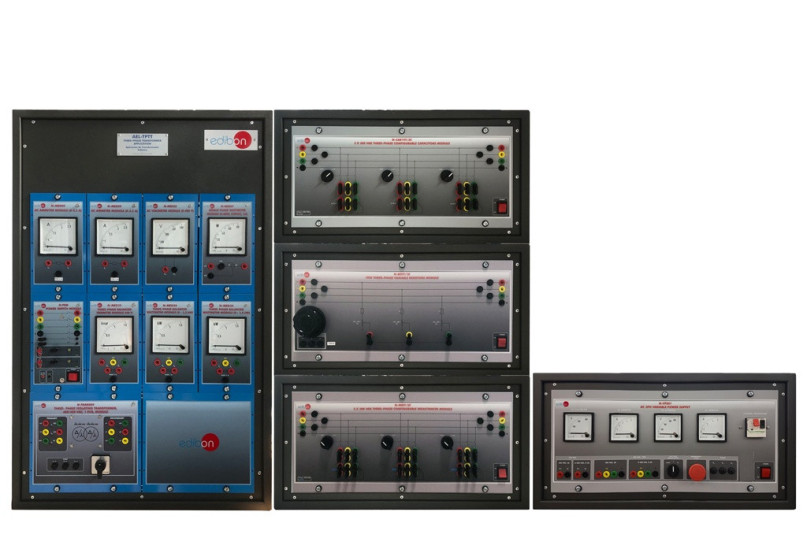# AEL-TPTT 三相变压器应用## 創新系統

The Three-Phase Transformer Application, "AEL-TPTT", has been designed by EDIBON to allow the students learn how to carry out different three-phase transformer tests and how they work.

## 一般說明

The Three-Phase Transformer Application, "AEL-TPTT", has been designed by EDIBON to allow the students learn how to carry out different three-phase transformer tests and how they work.

With this application the user will study the basic operating principles of the three-phase transformers as the practical realization of different tests to determinate the most relevant parameters of the machine. The equivalent circuit will be determinate as its power losses and global efficiency. Also, the application includes resistive, inductive and capacitive loads to carry out different connections and determinate the voltage drops of the transformer due to this loads.

The "AEL-TPTT" application includes all necessary modules to realize the practical exercises mentioned previously, even other exercises designed by the user. Between this modules a power supply, transformer, ammeters, voltmeters, multimeters an resistive, inductive and capacitive loads are included. Optionally, another transformer can be coupled in order to study the parallel connection of both of them and their mutual behavior.

## 練習和指導練習

### 手册中包含的指导实践练习

1. Wiring the three-phase transformer according with different configurations.
2. Calculation of transformer ratio according with different configurations.
3. Open circuit test.
4. Short circuit test.
5. Determination of equivalent circuit.
6. Drop voltage with resistive load.
7. Drop voltage with inductive load.
8. Drop voltage with capacitive load.
9. Study the efficiency of the transformer.
10. Determination of load which allows the maximum efficiency.

Some practical exercises possibilities in order to study transformers in parallel connection:

1. Objectives of transformers connected in parallel and conditions.
2. Study of equivalent circuit of the coupling with load.
3. Study of equivalent circuit of the coupling without load.
4. Analyzing of currents varying the power, short-circuit voltage and transformer ratio.

### 与该单位进行更多实际操作

1. Several other exercises can be done and designed by the user.

## 可用的类似设备

#### 質量#### 售後服務